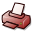# Strongly constrained maximal subgroups and Sims order bounds for finite almost simple linear and unitary groups

## DSpace Repository

 URI: Dokumentart: Dissertation Date: 2017-07-07 Language: English Faculty: 7 Mathematisch-Naturwissenschaftliche Fakultät Department: Mathematik Advisor: Knapp, Wolfgang (Prof. Dr.) Day of Oral Examination: 2017-05-19 DDC Classifikation: 510 - Mathematics Keywords: Gruppentheorie Other Keywords:Sims conjectureAschbacher theoremstrongly constrained maximal subgroupsfinite almost simple linear groupfinite almost simple unitary grouplargest normal p-subgroupcentralizer License: Order a printed copy:Print-on-Demand Show full item record

### Abstract:

The main topics of this thesis are the strongly constrained maximal subgroups of the finite almost simple linear and unitary groups, i.e. maximal subgroups whose generalized Fitting subgroup coincides with the largest normal p-subgroup for a prime p. The motivation to consider this particular class of local maximal subgroups arises by the Sims conjecture, a theorem of Wielandt and the O'Nan-Scott theorem: In the middle of the 1960s, Sims conjectured that for a finite primitive permutation group G the order of a point stabilizer is bounded by a function f in terms of an arbitrary non-trivial subdegree d of G. A function f which satisfies the conditions of the conjecture of Sims (for a collection H of finite primitive permutation groups) is called a Sims order bound (for H). By a theorem of Wielandt, one can establish an explicit Sims order bound for the collection consisting of the finite primitive permutation groups whose point stabilizers are not strongly constrained. Furthermore, using the O'Nan-Scott theorem, one may show that an explicit Sims order bound f can be determined if an explicit Sims order bound for the collection consisting of the finite almost simple primitive permutation groups is known. So, to obtain an explicit Sims order bound f it is sufficient to investigate the case of finite almost simple primitive permutation groups which have a strongly constrained point stabilizer. In this thesis we consider finite almost simple groups with linear or unitary socle. In Chapter 2 we determine the pairs (G,M) where G is a finite almost simple linear or unitary group and M a strongly constrained maximal subgroup of G. In particular, we classify all strongly constrained maximal subgroups of the finite almost simple linear and unitary groups. Here, we use the classification of the maximal subgroups of the finite almost simple classical groups, obtained by Aschbacher, Kleidman, Liebeck, Bray, Holt and Roney-Dougal. Often, we determine also additional facts, such as the determination of the largest normal p-subgroup O_{p}(M) of the strongly constrained maximal subgroup M of G (p the appropriate prime), or the determination of the centralizer of O_{p}(M) in G. Using the classification obtained in Chapter 2, in Chapter 3 we determine an explicit Sims order bound for the collection consisting of the finite almost simple primitive permutation groups whose socle is isomorphic to a projective special linear or unitary group and which have a strongly constrained point stabilizer. By this result, we deduce that the Wielandt order bound wdt(d)=d!((d-1)!)^{d} is a Sims order bound for the collection consisting of the finite primitive permutation groups which are almost simple linear or unitary groups.